What is the value of 123² - 23²?

A:B:C:D:E:What is the value of (2019 − (2000 − (10−9))) − (2000 − (10 − (9 − 2019))) (2019 − (2000 − (10−9))) − (2000 − (10 − (9 − 2019))) ?

A:B:C:D:E:3. Used in measuring the width of a wire, one mil is equal to one thousandth of an inch. An inch is about 2.5 cm.

Which of these is approximately equal to one mil?

A: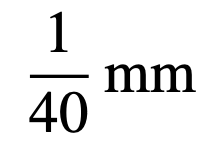B: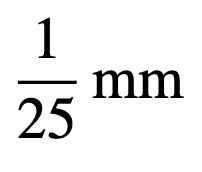C: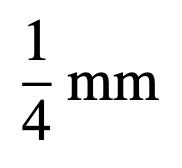D: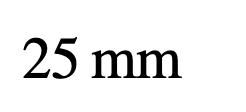E: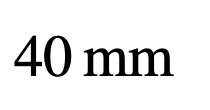4. For how many positive integer values of n is 2+2n prime?

A:B:C:D:E:5. Olive Green wishes to colour all the circles in the diagram so that, for each circle, there is exactly one circle of the same colour joined to it.

What is the smallest number of colours that Olive needs to complete this task?

A:B:C:D:E:6. Each of the factors of 100 is to be placed in a 3 by 3 grid, one per cell, in such a way that the products of the three numbers in each row, column and diagonal are all equal. The positions of the numbers 1, 2, 50 and x are shown in the diagram.

What is the value of x?

A:B:C:D:E:7. Lucy is asked to choose p, q, r and s to be the numbers 1, 2, 3 and 4, in some order, so as to make the value of

as small as possible.

What is the smallest value Lucy can achieve in this way?

A: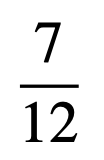B: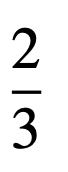C: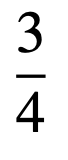D: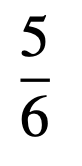E: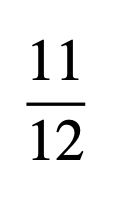8. The number 𝜲 is the solution to the equation 3(³ˣ) = 333.

Which of the following is true?

A:B:C:D:E:9. A square of paper is folded in half four times to obtain a smaller square. Then a corner is removed as shown.

Which of the following could be the paper after it is unfolded?

A: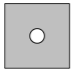B: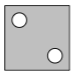C: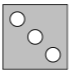D: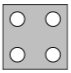E: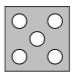10. Which of the following five values of n is a counter example to the statement in the box below?

A:B:C:D:E:11.

A:B:C:D:E:12. A circle with radius 1 touches the sides of a rhombus, as shown. Each of the smaller angles between the sides of the rhombus is 60∘.

What is the area of the rhombus?

A: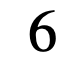B: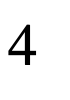C: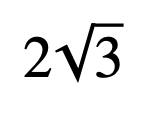D: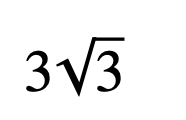E: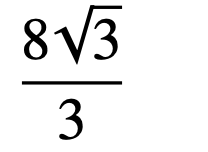13. Anish has a number of small congruent square tiles to use in a mosaic. When he forms the tiles into a square of side n, he has 64 tiles left over. When he tries to form the tiles into a square of side n + 1, he has 25 too few.

How many tiles does Anish have?

A:B:C:D:E:14. One of the following is the largest square that is a factor of 10!. Which one?

Note that, n! = 1 × 2 × 3 × ∙∙∙× ( n − 1 ) × n.

A:B:C:D:E:15. The highest common factors of all the pairs chosen from the positive integers Q, R and S are three different primes.

What is the smallest possible value of Q + R + S?

A:B:C:D:E:Back to the top